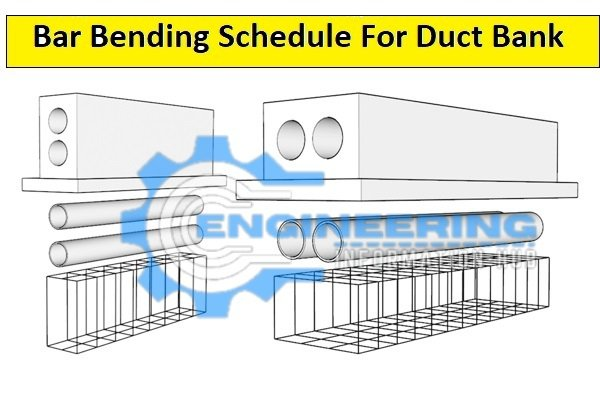Bar Bending Schedule

# Bar Bending Schedule For Duct Bank

## Bar Bending Schedule For The Duct Bank

The duct bank is also called the conduit bank. duct bank is construction on that location where the electrical cable is passing under any road. in the duct bank, we use PVC conduit or pipe and also use rebar in construction duct bank. Bar Bending Schedule For Duct Bank,How To Calculate The Bar Bending Schedule For Duct Bank| Bar Bending Schedule For Duct Bank| Calculate The Bar Bending Schedule For Duct Bank

### Bar Bending Schedule For The Duct Bank

Bar bending schedule means we find the rebar by using the duct bank cutting length of rebar diameter of the rebar and weight of the rebar. we find also the fixing of the rebar location and spacing of the rebar in the duct bank.

Now we calculate the bar bending schedule for the duct bank with the help of the given data. given data is only, for example, to explain the all steps, and this example to get very easy all steps and correct information.

### Given Data

length of the duct bank=8m

width of The duct bank = 1870mm=1.870m

height of duct bank=480mm

diameter of main bar=16mm

diameter of distribution bar=16mm

dia meter of sidebar =12mm

Spacing of all bar=150mm

Concrete Cover of all bar=75mm

### Solution

Length of the main bar/bottom bar

weight of the main bar/bottom bar

length of distribution bar/top bar/long bar

Weight of distribution bar/top bar/long bar

length of sidebar

weight of sidebar#### Length of the main bar

Cutting length of main bar= total width of duct bank-2C.C+2 bends

Cutting length of main bar=1870-2(75)+2(20d)

Cutting length of main bar/bottom bar=1870-150+2(20×16)=2360mm=2.360m

#### Number of bars

Number of bar=total length /spacing +1

Number of bar=8000/150+1=54.33=55nos

### The total length of the main bar

Total length of main bar=Numbers of bar × cutting length

Total length of main bar= 55 × 2.360=129.8m

### Weight of the main bar

Weight of main bar=d²/162.62×total length

Weight of main bar=16²/162.62×129.3=203.546kg=204kg

Same length and the same weight as the main bottom bar and main top bar

Total weight =Main bar bottom+Main bar top

Total weight =204kg+204kg=408KG

### Length of distribution bar /long bar

Cutting length of distribution bar=total length of duct bank -2C.C+2 bends

Cutting length of distribution bar=8000-2(75) +2(20d)

Cutting length of distribution bar=8000-150 +2(20×16)=8490m=8.490m

### Number of distribution bar

Number of distribution bar=total width /spacing +1

Number of distribution bar=1870/150+1=13.466=14nos

### Total length of distribution bar

Total Length of distribution bar=Number of bar × cutting length

Total Length of distribution bar=14 × 8.490=118.86m

### Weight of distribution bar

Weight of distribution bar=d²/162.62×total length

Weight of distribution bar= 16²/162.62×118.86=187.112kg=188kg

Same length and the same weight as the distribution bar bottom and top bar

Total weight =distribution bar bottom+distribution bar top

Total weight =188+188=376kg

Total weight of 16mm bar=408+376=784kg

### Length of sidebar/straight bar

Cutting length of sidebar=total length of duct bank -2C.C

Cutting length of sidebar=8000-2(75)=7850mm=7.850m

### Number of sidebars

Number of sidebar=2

### The total length of the Sidebar

The total length of Sidebar=Number of bars × cutting length

The total length of the Sidebar=2 × 7.850=15700mm=15.700m

### Weight of sidebar

Weight of sidebar=d²/162.62×total length

Weight of sidebar=12²/162.62×15.700=13.902kg=14kg